# How to Calculate and Solve for Pressure and Initial Compressibility Factor Ratio of a Reservoir Fluid Flow | The Calculator Encyclopedia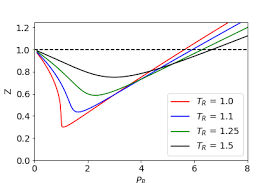The image above represents pressure and initial compressibility factor ratio (P/Z Ratio).

To compute for the pressure and initial compressibility factor ratio, four essential parameters are needed and these parameters are initial pressure (Pi), Initial Compressibility Factor (Zi), Cumulative Gas Production (GP) and initial gas in place (G).

The formula for calculating the P/Z Ratio:

P/Z = Pi / Zi (1 – Gp / G)

Where;

P/Z = P/Z Ratio
Pi = Initial Pressure
Zi = Initial Compressibility Factor
GP = Cumulative Gas Production
G = Initial Gas in Place

Let’s solve an example;
Given that the initial pressure is 20, the initial compressibility factor is 28, the cumulative gas production is 30 and the initial gas in place is 34.
Find the pressure and initial compressibility factor ratio (P/Z Ratio)?

This implies that;

Pi = Initial Pressure = 20
Zi = Initial Compressibility Factor = 28
GP = Cumulative Gas Production  = 30
G = Initial Gas in Place = 34

P/Z = Pi / Zi (1 – Gp / G)
P/Z = 20 / 28 (1 – 30 / 34)
P/Z = 20 / 28 (1 – 0.88)
P/Z = 20 / 28 (0.117)
P/Z = 0.714(0.117)
P/Z = 0.084

Therefore, the P/Z Ratio is 0.084.

Calculating for Initial Pressure when P/Z Ratio, Initial Compressibility Factor, Cumulative Gas Pressure and Initial Gas In Place is Given.

Pi = P/Z x Zi / (1 – Gp / G)

Where;

Pi = Initial Pressure
P/Z = P/Z Ratio
Zi = Initial Compressibility Factor
GP = Cumulative Gas Production
G = Initial Gas in Place

Let’s solve an example;
Given that the P/Z Ratio is 50, the initial compressibility factor is 18, the cumulative gas production is 25 and the initial gas in place is 32.
Find the initial pressure ?

This implies that;

P/Z = P/Z Ratio = 50
Zi = Initial Compressibility Factor = 18
GP = Cumulative Gas Production = 25
G = Initial Gas in Place = 32

Pi = P/Z x Zi / (1 – Gp / G)
Pi = 50 x 18 / (1 – 25 / 32)
Pi = 900 / (1 – 0.78125)
Pi = 900 / (0.21875)
Pi = 4114.28

Therefore, the Initial Pressure is 4114.28.

Calculating for Initial Compressibility Factor when P/Z Ratio, Initial Pressure, Cumulative Gas Pressure and Initial Gas In Place is Given.

Zi = Pi (1 – Gp / G) / P/Z

Where;

Zi = Initial Compressibility Factor
Pi = Initial Pressure
P/Z = P/Z Ratio
GP = Cumulative Gas Production
G = Initial Gas in Place

Let’s solve an example;
Given that the P/Z Ratio is 40, the initial pressure is 18, the cumulative gas production is 15 and the initial gas in place is 22.
Find the initial compressibility factor ?

This implies that;

Pi = Initial Pressure = 18
P/Z = P/Z Ratio = 40
GP = Cumulative Gas Production = 15
G = Initial Gas in Place = 22

Zi = Pi (1 – Gp / G) / P/Z
Zi = 18 (1 – 15 / 22) / 40
Zi = 18 (1 – 0.681) / 40
Zi = 18 (0.319) / 40
Zi = 5.742 / 40
Zi = 0.1435

Therefore, the initial compressibility factor is  0.1435.

Calculating for Cumulative Gas Pressure when P/Z Ratio, Initial Pressure, Initial Compressibility Factor and Initial Gas In Place is Given.

Gp = G (P/Z x Zi / Pi) + 1

Where;

GP = Cumulative Gas Production
P/Z = P/Z Ratio
Zi = Initial Compressibility Factor
Pi = Initial Pressure
G = Initial Gas in Place

Let’s solve an example;
Given that the P/Z Ratio is 46, the initial pressure is 32, the initial compressibility factor is 19 and the initial gas in place is 29.
Find the cumulative gas production ?

This implies that;

P/Z = P/Z Ratio =46
Zi = Initial Compressibility Factor = 19
Pi = Initial Pressure = 32
G = Initial Gas in Place = 29

Gp = G (P/Z x Zi / Pi) + 1
Gp = 29 (46 x 19 / 32) + 1
Gp = 29 (874 / 32) + 1
Gp = 29 (27.3125) + 1
Gp = 792.0625 + 1
Gp = 793.0625

Therefore, the Cumulative gas pressure is 793.0625.

Calculating for Initial Gas In Place when P/Z Ratio, Initial Pressure, Initial Compressibility Factor and Cumulative Gas Pressure is Given.

G = (Gp – 1) (P/Z x Zi / Pi)

Where;

G = Initial Gas in Place
GP = Cumulative Gas Production
P/Z = P/Z Ratio
Zi = Initial Compressibility Factor
Pi = Initial Pressure

Let’s solve an example;
Given that the P/Z Ratio is 42, the initial pressure is 22, the initial compressibility factor is 9 and the cumulative gas production is 14.
Find the initial gas in place ?

This implies that;

GP = Cumulative Gas Production = 14
P/Z = P/Z Ratio = 42
Zi = Initial Compressibility Factor = 9
Pi = Initial Pressure = 22

G = (Gp – 1) (P/Z x Zi / Pi)
G = (14 – 1) (42 x 9 / 22)
G = (13) (378 / 22)
G = (13) (17.18)
G = 223.36

Therefore, the initial gas in place is 223.36.

Nickzom Calculator – The Calculator Encyclopedia is capable of calculating the P/Z Ratio.

To get the answer and workings of the P/Z Ratio using the Nickzom Calculator – The Calculator Encyclopedia. First, you need to obtain the app.

You can get this app via any of these means:

To get access to the professional version via web, you need to register and subscribe for NGN 1,500 per annum to have utter access to all functionalities.
You can also try the demo version via https://www.nickzom.org/calculator

Apple (Paid) – https://itunes.apple.com/us/app/nickzom-calculator/id1331162702?mt=8
Once, you have obtained the calculator encyclopedia app, proceed to the Calculator Map, then click on Petroleum under EngineeringNow, Click on Reservoir Fluid Flow under Petroleum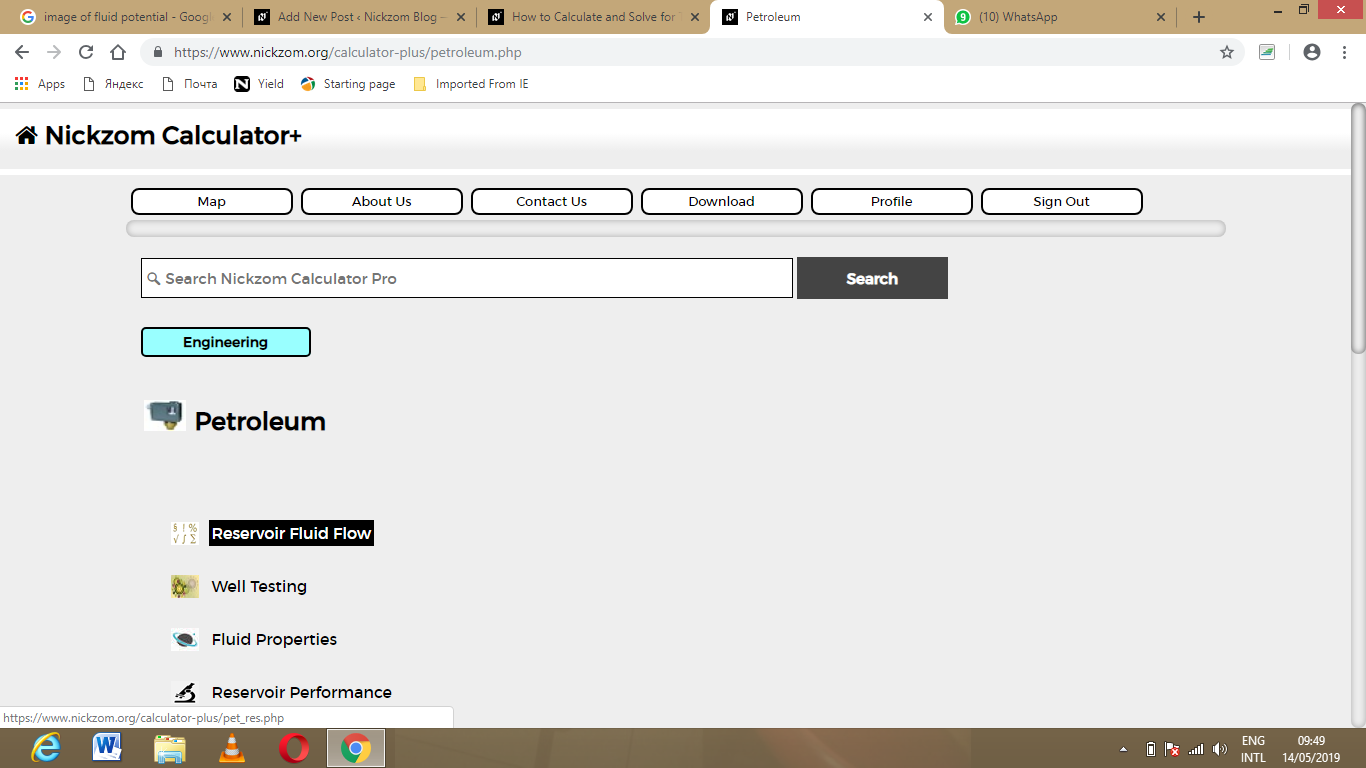Now, Click on P/Z Ratio under Reservoir Fluid Flow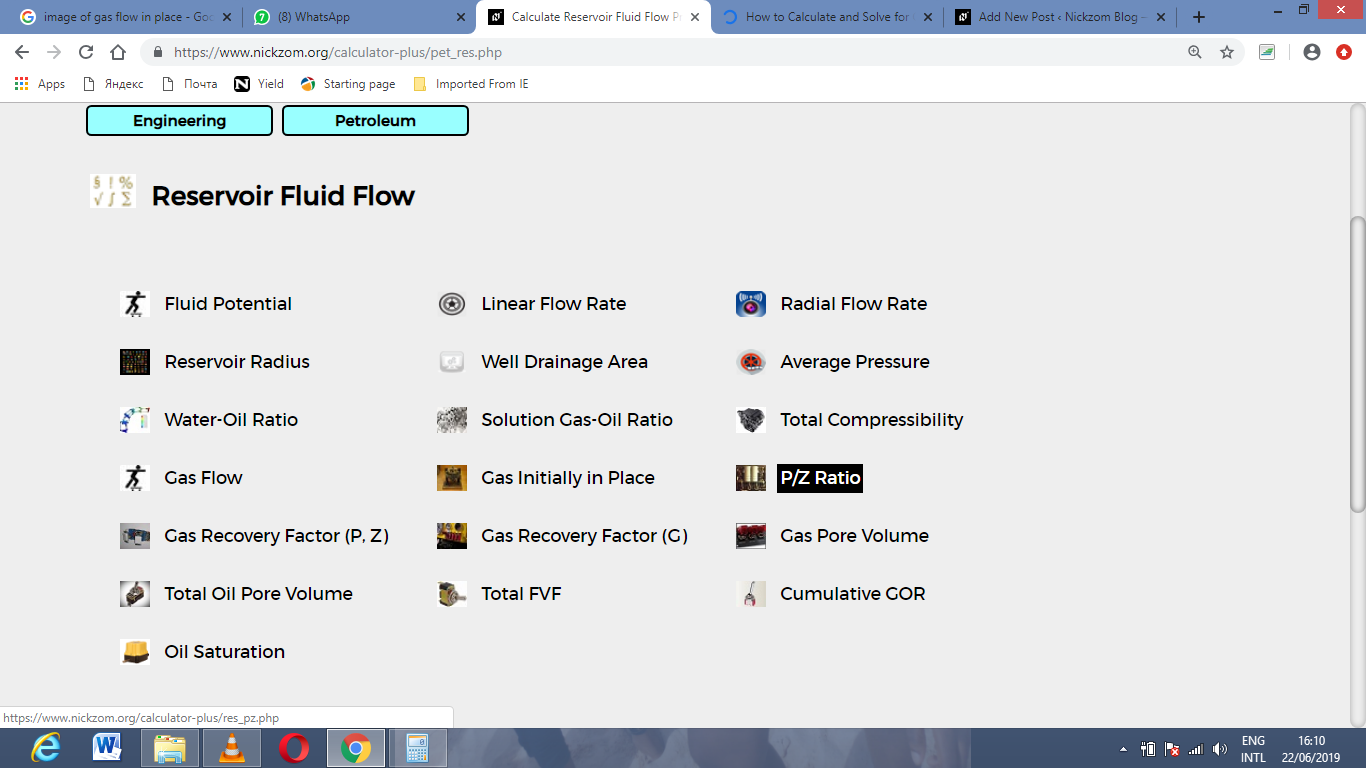The screenshot below displays the page or activity to enter your value, to get the answer for the P/Z Ratio according to the respective parameter which are the initial pressure (Pi), Initial Compressibility Factor (Zi), Cumulative Gas Production (GP) and initial gas in place (G).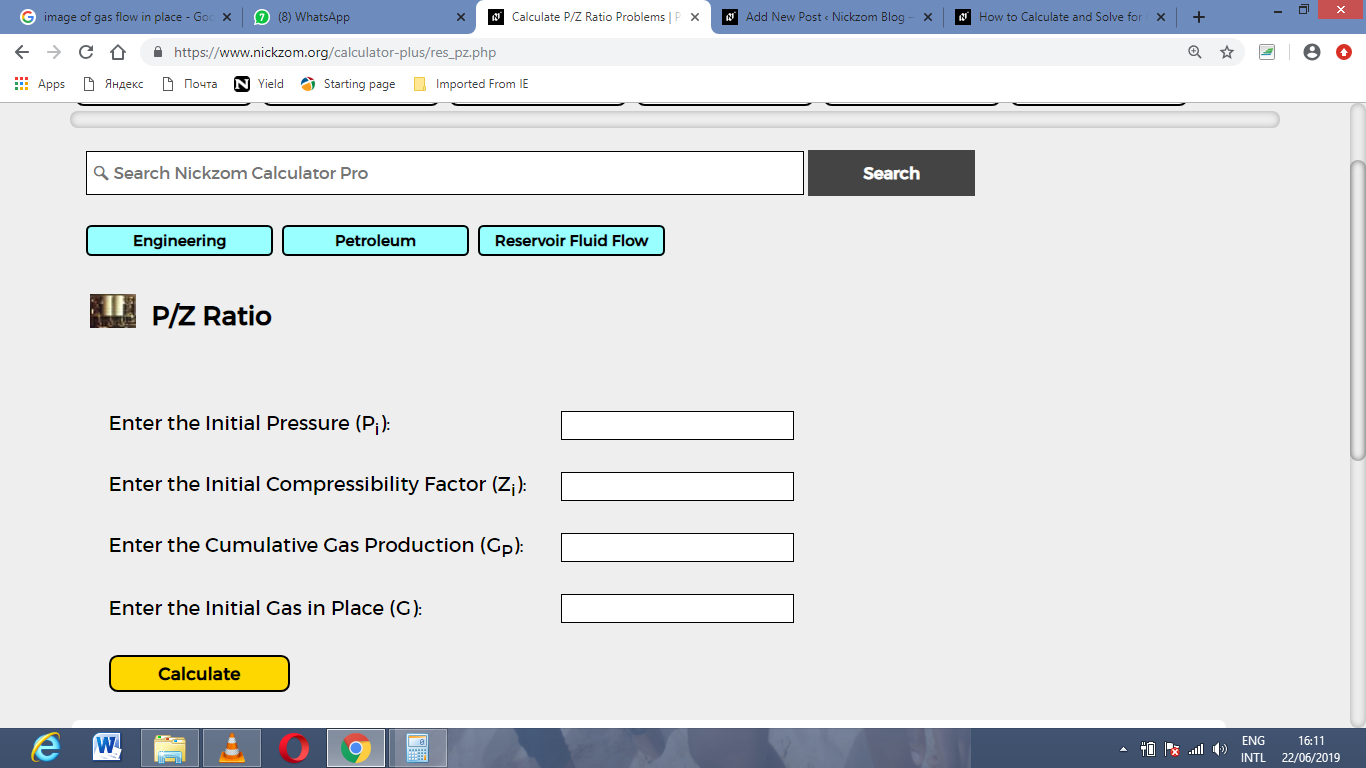Now, enter the value appropriately and accordingly for the parameter as required by the initial pressure (Pi) is 20, Initial Compressibility Factor (Zi) is 28, Cumulative Gas Production (GP) is 30 and initial gas in place (G) is 34.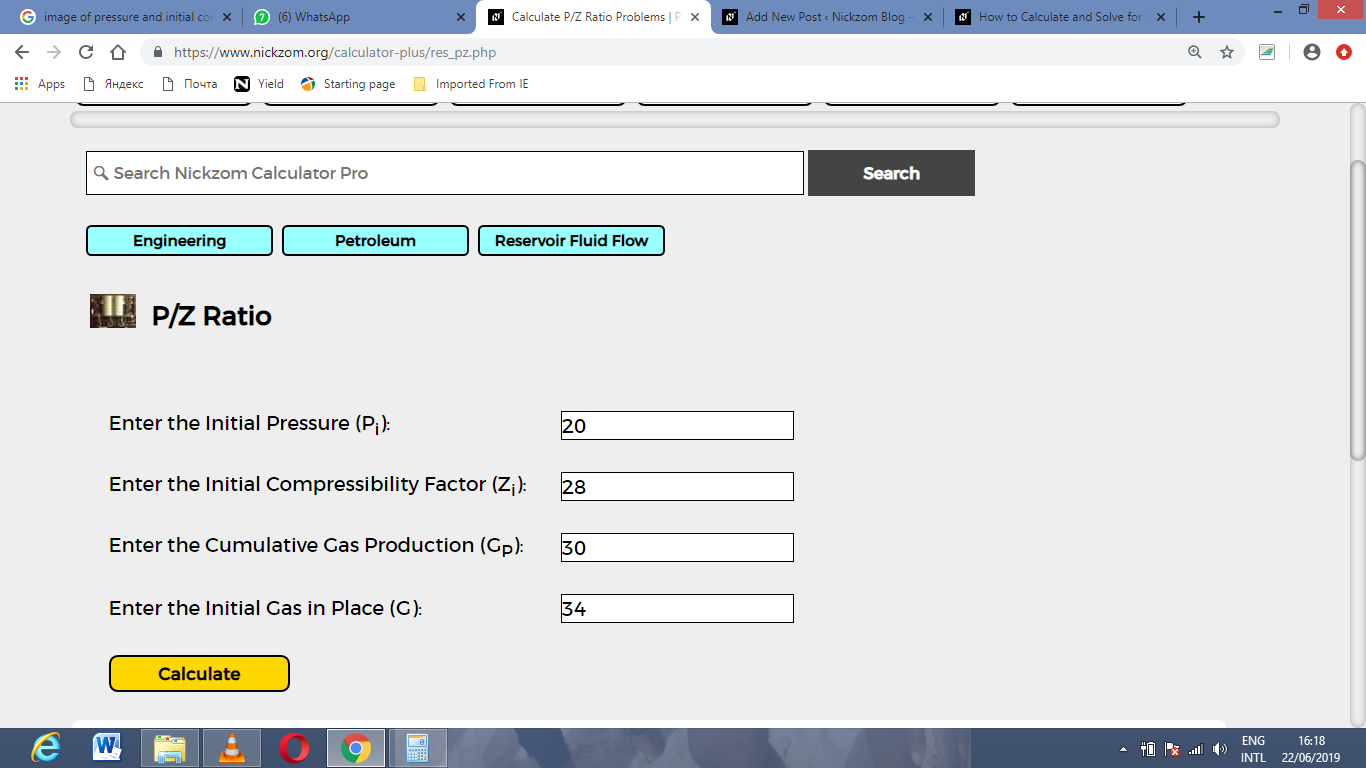Finally, Click on Calculate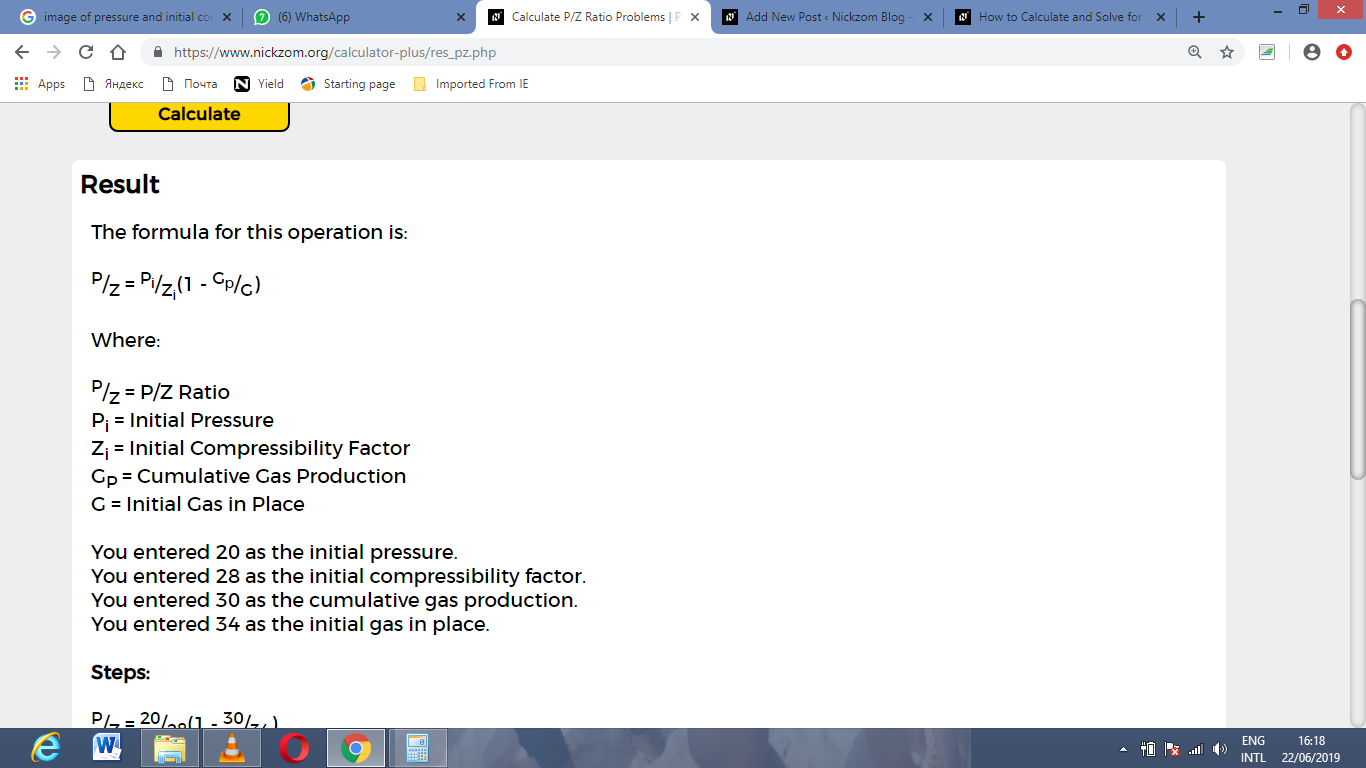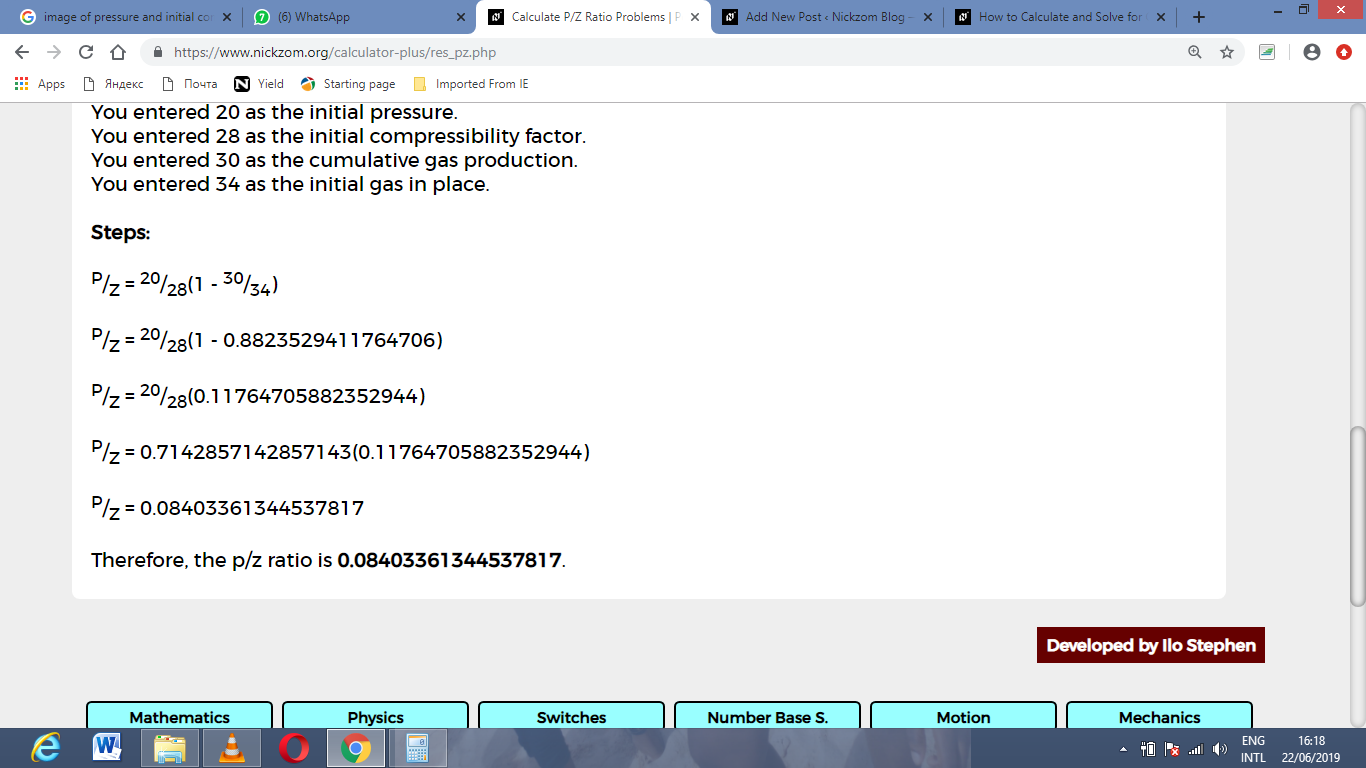As you can see from the screenshot above, Nickzom Calculator– The Calculator Encyclopedia solves for the P/Z Ratio and presents the formula, workings and steps too.